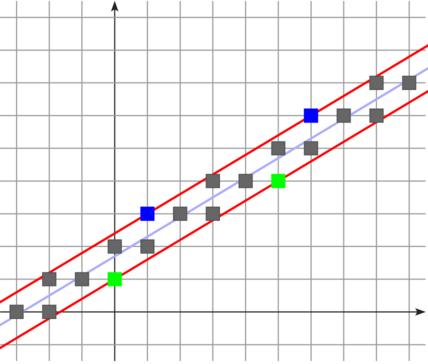DGtal  1.3.beta
Patterns, digital straight lines and subsegments
Author(s) of this documentation:
Jacques-Olivier Lachaud

Part of the Arithmetic package.

This part of the manual describes digital straightness from a combinatoric and arithmetic point of view. It tells you how to create patterns, digital straight lines in the first quadrant, and how to compute subsegments in sublinear time (algorithms SmartDSS [Said, Lachaud, Feschet 2009 :  ] and ReversedSmartDSS [Said, Lachaud 2011 : ]). Full proofs and details on these two algorithms are given in [Lachaud, Said 2013 :  ].

# Standard digital straight lines and patterns

A standard digital straight line in 2D (DSL) is the following subset of $$Z^2$$:

$$\{(x,y) \in Z^2, \mu \le a x - by < \mu + |a| +|b|-1 \}$$

where the integers $$(a,b,\mu)$$, $$gcd(a,b)=1$$, are called the characteristics of the straight line [ Reveilles  , Debled and Reveilles  ].

It is well known that this set of digital points is a simple 4-connected curve. Here is a representation of the straight line (3,5,-5).Standard Digital Straight Line of characteristics (3,5,-5)

The slope of the DSL is $$a/b$$, while $$\mu$$ is the shift at origin. The remainder of the DSL is the value $$ax-by$$. Upper leaning points have remainer $$\mu$$ while lower leaning points have value $$\mu + |a| +|b|-1$$.

# Patterns

## Definition of patterns

A pattern of slope $$a/b$$ is the freeman chaincode between two consecutive upper leaning points of any DSL with slope $$a/b$$.

Patterns are specific subsegments of digital straight lines (DSL). They depend only on the slope of the DSL, i.e. the irreducible fraction $$a/b$$.

## Creating patterns in DGtal

Package Arithmetic provides the class Pattern to represent a pattern. Patterns are instantiated either by a fraction or two integers p and q. They are therefore parameterized by the type of irreducible fraction you wish to use (see Irreducible fraction, continued fractions). You may define patterns as follows:

pattern.cpp
typedef DGtal::int32_t Quotient;
typedef LighterSternBrocot<Integer, Quotient, StdMapRebinder> SB; // the type of the Stern-Brocot tree
typedef SB::Fraction Fraction; // the type for fractions
typedef Pattern<Fraction> MyPattern; // the type for patterns
DGtal::int32_t p = atoi( argv[ 1 ] );
DGtal::int32_t q = atoi( argv[ 2 ] );
MyPattern pattern( p, q );

To get the freeman chaincode of the pattern, you may use the methods Pattern::rE and Pattern::rEs.

bool sub = ( argc > 3 ) && ( std::string( argv[ 3 ] ) == "SUB" );
std::cout << ( ! sub ? pattern.rE() : pattern.rEs( "(|)" ) ) << std::endl;
$./examples/arithmetic/pattern 11 17 0010010100101001010010100101$ ./examples/arithmetic/pattern 11 17 SUB
((00|1)|(0|0101)(0|0101)(0|0101)(0|0101)(0|0101))


## Recursive properties of patterns

Recursive definitions of pattern are related to the continued fraction of the slope. We choose here to present the Berstel recursive definition. If $$z=p/q=[u_0;u_1,\ldots,u_n]$$ is the slope of the pattern, the pattern may be obtained from the following recursive formulas:

$$E(z_{-1})=1, E(z_{0})=0, E(z_{2k+1})=E(z_{2k})^{u_{2k+1}} E(z_{2k-1}), E(z_{2k})=E(z_{2k-2}) E(z_{2k-1})^{u_{2k}}.$$

Let us look again at the pattern of 11/17. First, the convergents are:

\$ ./examples/arithmetic/convergents 11 17
z = [0,1,1,1,5]
z_0 = 0 / 1
z_1 = 1 / 1
z_2 = 1 / 2
z_3 = 2 / 3
z_4 = 11 / 17

E(0/1) = 0
E(1/1) = E([0;1]) = E(0/1)^1 E(z_-1) = 0.1
E(1/2) = E([0;1,1]) = E(0/1) E(1/1)^1 = 0.01
E(2/3) = E([0;1,1,1]) = E(1/2)^1 E(1/1) = 001.01
E(11/17) = E([0;1,1,1,5]) = E(1/2) E(2/3)^5 = 001.(00101)^5


Which is exactly the sought pattern.

There are several methods to compute the Bézout vector of a pattern or the coordinates of its upper and lower leaning points (see reference of class Pattern).

# Digital straight lines and subsegments

## Creating a digital straight line

For now, you may only instantiate digital straight lines whose slope is in the first quadrant. If $$(a,b,\mu)$$ are the characteristics of the line, then $$a \ge 0, b \ge 0$$.

...
#include "DGtal/arithmetic/StandardDSLQ0.h"
...
typedef ... Fraction;
typedef StandardDSLQ0<Fraction> DSL;
...
DSL D( 3, 5, -5 );

## A DSL is a sequence of points in the digital plane

A DSL provides the function operator StandardDSLQ0::operator(), taking a Point p and returning 'true' iff p belongs to the set of points of the DSL. It is indeed a model of concepts::CPointPredicate.

The Point type is PointVector<2,Fraction::Integer>.

You may visit the DSL from left to right with iterators of type StandardDSLQ0::ConstIterator. You just precise the starting point with StandardDSLQ0::begin and the point after the last with StandardDSLQ0::end.

You can compare if a point precedes another point (to the left) on the DSL with methods StandardDSLQ0::before and StandardDSLQ0::beforeOrEqual.

## Getting points on a DSL

For convenience, you have the following methods to determine points belonging to a DSL:

## Fast computation of subsegments

Given two points A and B on the DSL, you can determine the exact characteristics of the subsegment [A,B] in sublinear time with two algorithms: SmartDSS [Said, Lachaud, Feschet 2009 :  ] and ReversedSmartDSS [Said, Lachaud 2011 : ]. In fact, these algorithms return the DSL which covers [A,B] and whose characteristics are minimal.

• method StandardDSLQ0::smartDSS, given this DSL, A and B, returns this minimal DSL in time proportional to the sum of the quotients of the output DSL.
• method StandardDSLQ0::reversedSmartDSS, given this DSL, A and B, returns this minimal DSL in time proportional to the difference of depth between the input and the output slope.

You may have a look at the following programs to check these algorithms (the many variants come from the different choices for the algorithm and the fraction type):

DGtal::int32_t
boost::int32_t int32_t
signed 32-bit integer.
Definition: BasicTypes.h:72
Integer
Point::Coordinate Integer